Abstract: A hardware-oriented Gaussian random noise generation method for mean and variance is proposed. The traditional Monte Carlo method is used to realize the rapid conversion of uniformly distributed noise to identically distributed noise. The mapping function method is used to achieve a transition to reduce the amount of calculations at random. Experiments prove that this method has the advantages of fast and high precision.

Gaussian random noise has important value in signal analysis and processing. This article specifically discusses a hardware-oriented algorithm for rapid generation of Gaussian noise. The principle of the algorithm is to transform the uniform noise into Gaussian random noise through the mapping table method. This method occupies small memory, fast calculation speed and high precision. Experiments show that this method can add Gaussian noise to video images in real time.

figure 1

The principle block diagram is shown in Figure 1. The left half of Figure 1 is the part of uniform noise generation, adopting the modulus remainder method, relying on the existing K random number seeds to continuously generate new random numbers and output them to the outside. The right half of Figure 1 uses the output value of the left half to generate the mapping table address, and outputs the result obtained after the table lookup, which is the required Gaussian noise value result.

1 Generate uniformly distributed noise

Let x (1), ..., x (k) be the given k random numbers, evenly distributed in (-X, X), and independent of each other. The k + 1th random number is obtained by adding x (1) and x (k) according to modulo X: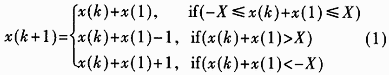That is, x (k + 1) is still a random number between (-X, X). In the next operation, move x (k + 1) to the position of x (k), xk) to x (k-1), ..., x (2) to x {1}, and then do the modulo X operation, add to get x (k + 2), so loop, generate sequence {xn}.

First discuss the above modular X operation. For processors using complement operations, as long as X is taken as the processor word length, the usual addition is modulo addition without considering carry.

Then use the data structure to achieve the above cycle mechanism. Set two pointers: head pointer head and tail pointer tail. After calculating the noise value, both pointers must move down one position. When it reaches the end of the sequence, it refers to the head of the sequence again, that is, it is calculated by adding 1 modulo K: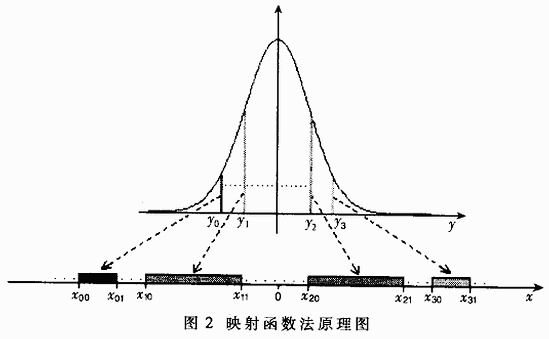For the convenience of calculation, choose K as 256. If head and lnil pointers are used as relative offset addresses, 1 byte can be used for storage. If the overflow is not considered after the addition operation, it is equivalent to the addition of modulo 256 operation.

2 Mapping Gaussian noise

In order to quickly generate Gaussian noise from uniform noise, the mapping function method is used. The algorithm principle is shown in Figure 2.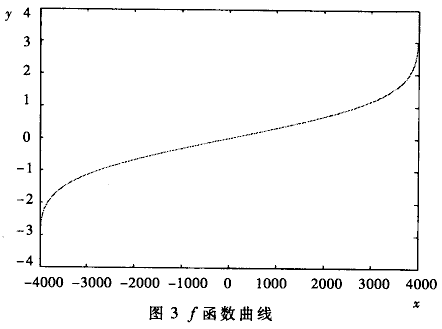The independent variable y of the normal distribution function is reasonably discretized to obtain a series of function values â€‹â€‹to form a sequence. Then map any number yi in this sequence to a certain inter-cell segment [xi0, xi1] on the number axis x, where â€–xi1ï¼xi0â€– = yi. Take all the values â€‹â€‹in the series to get a series of inter-cells. Connect all the cells in sequence, and place the midpoint at the zero point of the number axis to form the value interval [-x, x] of the variable x. By establishing a mapping relationship between the cells on the number axis x and the y sequence, it can be proved that if the sampling interval is small enough, the random distribution in the x domain will correspond to the Gaussian distribution in the y domain.

This mapping relationship can be expressed functionally as y = f (x).

Among them, x follows a uniform distribution in the interval (-X, X), while y follows a Gaussian distribution with mean u and variance Ïƒ. The f function curve is shown in Figure 3.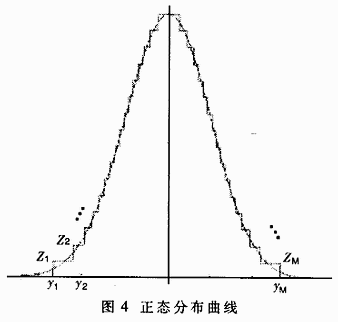When the algorithm is implemented, the Gaussian distribution value corresponding to y is quantized, and a continuous memory area [0, 2X] is allocated to x. The value of (offset address-X) of each memory unit represents the size of x value, The content of the unit stores the D value corresponding to the unit. This memory area is the required mapping lookup table. This table is versatile and can be calculated in advance. It can be used directly when needed, without further calculation.

For the (0,1) normal distribution, zi in Figure 4 is less than 1, which has no practical significance, so it is enlarged by 200 times;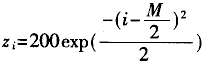Then the original mapping table is described as: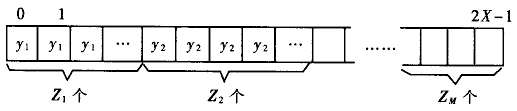The original mapping table is relatively large, and it is roughly sampled in actual use. The quantization of the Gaussian distribution value corresponding to y does not use the standard ladder form in Figure 4, but uses the following coarse sampling form:

Result mapping table [k] = original mapping table [k Â· d + d / 2] (2)

Among them: sampling interval d = âˆ‘yi / M. The quantization curve is shown in Figure 5.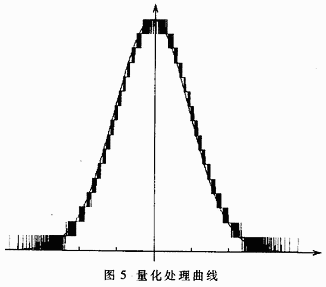It can be found from Figure 5 that the smaller parts on both sides are not cut off to 0 as in the ladder diagram, but allow non-zero values â€‹â€‹to occur with a small probability. Experiments prove that this method is better.

Considering the actual situation of the Gaussian distribution, and after experiments verifying that y only takes values â€‹â€‹between [-4, 4], satisfactory accuracy can be achieved. Figure 6 shows the curve of the effect of X size on noise accuracy, and the vertical axis is the average error.

It can be seen that when 2X is greater than 5500, the error reaches a very small steady state. In order to facilitate calculation, choose 2X = 213 = 8192, that is X = 212 = 4096.

Figure 7 shows the experimental results (mean value 120, variance 40): the dotted line is the standard Gaussian distribution curve, and the solid line is the noise histogram, which verifies the effectiveness of this method.

3 Precision discussion

This method mainly uses two tables: one is a table that generates uniform noise cyclically; the other is a mapping table. The values â€‹â€‹of these two tables can be calculated by the method introduced earlier, only the accuracy tradeoffs are discussed below.

The accuracy requirement of 256 seeds in the circular table is directly related to the size of the mapping table, and 8192 (8K) units are used for the mapping table. Therefore, the task of the circular table is to generate uniformly distributed random numbers in the interval [-4096, 4096]. Therefore, the seed sequences x (1), ..., x (256) in the circular table are evenly distributed in [-4096, 4096]. In order to facilitate calculation, the distribution of the seed sequence is adjusted between [-(2115-1), (215-1)], that is X = 215 = 32767. Therefore, a total of 16 bits including a 1-bit sign bit can be used to store the complement of the seed number, so that the modulo X addition operation can be realized by adding the complement of the carry. The upper 13 bits of the complement operation result or the right shift by 3 bits can be directly used as the offset address of the mapping table for subsequent table lookup operations, and can be evenly distributed within [-4096, 4096].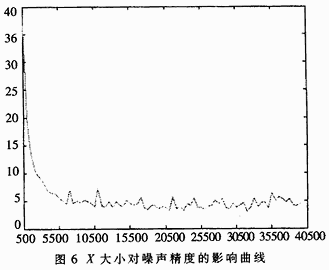Considering the actual situation, the variance of the Gaussian noise distribution to be sought is generally between (0, 100), and the mean is generally between [-255, 255]. The gray value of the actual image exists in the form of integer [0, 255],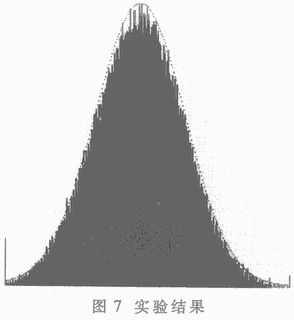Therefore, the accuracy of the noise can be controlled within an integer range, and the accuracy of the mapping table is sufficient as long as it reaches 0.01. The distribution of data in the mapping table is between [-4.00, 4.00]. If stored as an integer, the original data can be stored in 16-bit length units by multiplying by 128.

The table can also be modified on this basis, so that the mean and variance of the Gaussian distribution after the mapping are directly equal to the mean u and variance Ïƒ given by the user. The modification is relatively simple, replace each cell value y in the mapping table as follows:

y = (y * Ïƒ) >> 7 + u

The data in this table can be in the original code form or the complement form, depending on the specific needs.

The world premiere of the technical zone! ROHM has developed the power supply IC "BD372xx series" for high-quality audio. A practical guide for the purchase of home wireless routers. Understanding the circuit diagram and working principle of the audio. Talking about the "frequency response curve" in the audio. Deep dismantling report of the M0pro speaker: both internal and externalInteresting and informative information and technical dry goodsCreate your own personal electronic circleLock the latest course activities and technical live broadcast
Collect People collection
comment
Publish

related suggestion

var check_allow = "/d/Api/iscantalk.html"; var add_url = '/ d / article / write /'; function CheckLogin () {now_uid = ''; var ElecfansApi_checklogin = '/ webapi / passport / checklogin'; var logout_url = "{: U ('Login / logout')}"; var logout_url = 'http://bbs.elecfans.com/member.php?mod=logging&action=logout&refer=front'; \$ .get (ElecfansApi_checklogin, function (data, textStatus) {if (data! = "") {EchoLoginInfo (data); CheckEmailInfo (data); data = \$ .parseJSON (data); now_uid = data.uid; / * var login_content = 'write an article'+ data.username +'
Quit '; * / var login_content =' write an article'+ data.username +'
Set exit '; \$ (' # login_area '). Html (login_content); var win_width = \$ (window) .width (); if (win_width> 1000) {\$ ("# mine"). MouseDelay (200) .hover (function () {\$ ("# mymenu"). show ();}, function () {\$ ("# mymenu"). hide ();});}} else {var content = 'Login Registration'; \$ ('# login_area'). html (content); \$ (". special-login"). click (function (e) {\$ .tActivityLogin (); return false;});}});} \$ (function () {// comment ------------------------------- var comment = \$ ("# comment"); var comment_input = \$ ("# comContent"); // Submit comment click event interaction \$ ("# comSubmit2"). on ('click', function () {var content = comment_input.text (); // Empty input box comment_input. html (""). focus (); // Submit data to server \$ .ajax ({url: '/plus/arcComment.php', data: {aid: \$ ("# webID"). val (), dopost : 'apiPubComment', content: content}, type: 'post', dataType: 'json', success: function (data) {// Data format returned: if (data.status == "successed") {// Build temporary comment DOM var dom = ''; dom + = '
'; dom + =''; dom + ='
'; dom + ='

'+ data.data.username +' '; dom + ='

'; dom + =' '+ content +' '; dom + =' '; dom + =' just now '; dom + =' '; dom + =' '; // insert a temporary comment to the list \$ ("# comment ") .append (dom);} if (data.status ==" failed ") {// alert (data.msg); layer.msg (data.msg);}}}); return false;}); (function () {/ * * Insert single sign-on JS * / var setHost = 'https://passport.elecfans.com'; // Set domain name var script = document.createElement ('script'); script.type = 'text / javascript'; script.src = setHost + '/public/pc/js/t.passport.js'; script.setAttribute ("id", "sso_script"); script.setAttribute ("data-ssoSite", setHost); script.setAttribute ("data-ssoReferer", encodeURIComponent (location.href)); script.setAttribute ("data-ssoSiteid", "11"); var body = document.getElementsByTagName ("body"). item ( 0); body.appendChild (script);}) () / * * It is recommended to modify the style of the article without a picture * * / \$ (". Article .thumb"). Each (function () {if (\$ (this). find ('img'). attr ('src') == "") {\$ (this) .find ('img'). remove (); \$ (this) .parent (). css ('padding-left ',' 0px ');}}); / * Baidu share * / window._bd_share_config = {common: {bdText: '', // Custom share content bdDesc: '', // Custom share summary bdUrl: window.location.href, // Custom share URL address bdPic: ''} , share: [{"bdSize": 60, "bdCustomStyle": true}]} with (document) 0 [(getElementsByTagName ('head')  || body) .appendChild (createElement ('script')). src = 'http://bdimg.share.baidu.com/static/api/js/share.js?cdnversion=' + ~ (-new Date () / 36e5)]; var add_url = '/ d / article / write / '; // var check_allow = "{: U (' Api / iscantalk ')}"; var check_allow = "/ d / api / iscantalk"; var click_items_length = \$ ('. art_click_count '). length; if ( click_items_length> 0) {var id_str = ''; \$ ('. art_click_count'). each (function () {id_str + = \$ (this) .attr ('data-id') + ',';}) // var url = "{: U ('Api / getclickbyids')}"; var url = "/ d / api / getclickbyids"; var id_data = 'id_str =' + id_str; \$ .ajax ({url: url, data: id_data, type: 'post', dataType: 'json', success: function (re) {if (re.list.length> = 1) {var list = re.list; for (var i in list) {var t emp_id = list [i] ['id']; var temp_span = \$ (". art_click_count [data-id =" + temp_id + "]") temp_span.html (list [i] ['click']);}} }})} \$ ("# comContent"). click (function () {if (now_uid == '') {\$ .tActivityLogin (); return false;}}) \$ (function () {var follow_wrap = \$ ( ".author-collect"); var now_uid = "{\$ _super ['uid']}"; var face_src = "{\$ _super ['uface']}"; var getFollowNum = \$ (". followNum strong"). html (); // Follow \$ (window) .on ('click', '.author-collect', function () {if (now_uid == '') {\$ .tActivityLogin (); return false;} if ( \$ (this) .attr ('id') == 'follow') {\$ .post ('/ d / user / follow', {tuid: article_user_id}, function (data) {// Data format returned: if (data.status == "successed") {\$ (". followNum strong"). html (++ getFollowNum); follow_wrap.html ('followed'). attr ('id', 'cancelFollow'). css ( 'background', '# 999'); var follow_user = ''; \$ (' # follow_list '). append (follow_user);} if (data.status == "failed") {alert (data.msg);}});} else {// Unfollow if (\$ ( this) .attr ('id') == 'cancelFollow') {\$ .post ('/ d / user / cancelFollow', {tuid: article_user_id}, function (data) {// Data format returned: if (data .status == "successed") {follow_wrap.html ('Follow'). attr ('id', 'follow'). css ('background', '# f90'); \$ (". followNum strong"). html (-getFollowNum); \$ ('# follow_list .face'). each (function () {var target_uid = \$ (this) .attr ('data-uid'); if (target_uid == now_uid) {\$ ( this) .remove ();}})} if (data.status == "failed") {alert (data.msg);}}); return false;}}});});}); / * var myface = "{\$ _super ['uid'] | avatar}"; var myname = "{\$ _super ['username']}"; var article_id = {\$ article ['id']}; var article_user_id = {\$ article ['mid']}; // Article author ID \$ (function () {<notempty name = "clearnum"> // Reduce the number of reminders var count = parseInt (\$ ("# noticeCount"). html ()); count = count-{\$ clearnum}; \$ ("# noticeCount"). html (count); if ( count

Professional Iron Lamp manufacturer is located in China, including Iron Pendant Lamp,Iron Pendant Light,Metal Pendant Light, etc.

the types of lamps include pendant lamps, table & floor lamps, chandelier, Wall Lamp ,desk lamp and etc., the materials include steel, metal, fabric, crystal, glass, aryclic and so on.

We offer samples with new designs every month to update our showroom and well display in lighting exihibtions.
We can make samples for his own designs as per shop drawings even potential idea from customers.

Iron Pendant Lamp

Iron Lamp,Iron Pendant Lamp,Iron Pendant Light,Metal Pendant Light

Monike lighting , https://www.monikelight.com